# Fractions 3

Fractions can be challenging when taught in an abstract way. That’s why this unit invites learners to engage with fractions and mixed numbers in very visual and concrete ways using number lines, tape diagrams and area models. Students will learn different strategies to practise identifying and generating equivalent fractions.
This lesson includes 5 printable learning activities.

## Fractions on a Number Line 3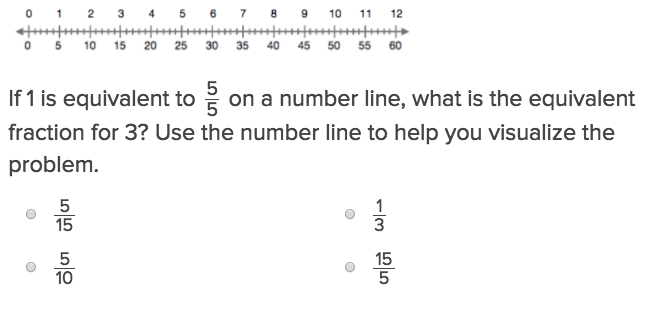Fractions on a Number Line 3 will help students practise this key year six skill.

## Unlike Denominator Fraction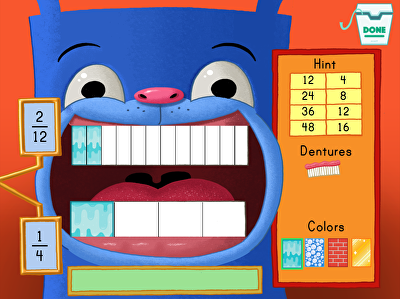This unlike denominator fraction game asks students to identify equivalent fractions and least common multiples. Then students add or subtract the new fractions to help Floyd get his new set of teeth at the dentist. practise with fractions and tape diagrams, such as the one used in this game, helps to establish a strong maths foundation in the higher grades. Fifth graders, in particular, can benefit from this type of practise.

## Fractions and Tape DiagramsBy using a tape diagram, students will be able to visualize fractions much more easily.

## Gem Mining: Converting Improper and Proper Fractions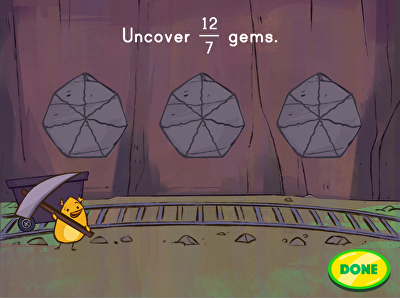Gem mining is a game that teaches converting improper to proper fractions. Students are tasked with helping Birdee uncover the gems in the mine by tapping on the rocks to match the mixed number shown. Students will practise the following fractions: halves, thirds, quarters, fifths, sixths, and eighths. Learning mixed numbers and improper fractions is a key maths skill typically covered in the year six.

## Mixed Numbers and Improper Fractions 3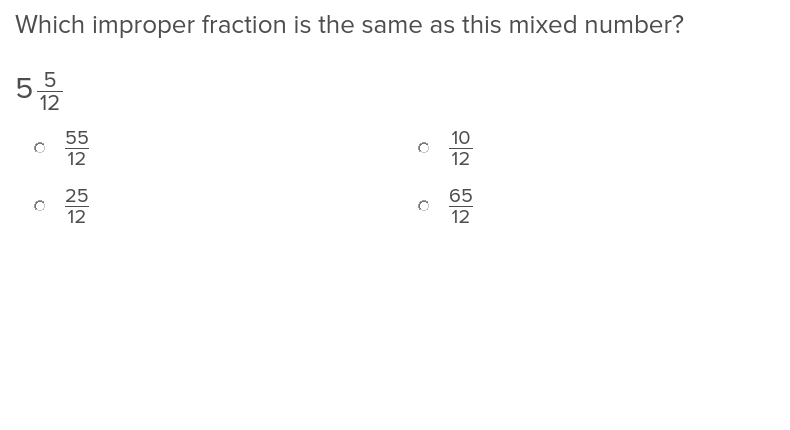After this final exercise in the series, students will be completely comfortable identifying and working with mixed numbers and improper fractions.

## Decimal Fractions 2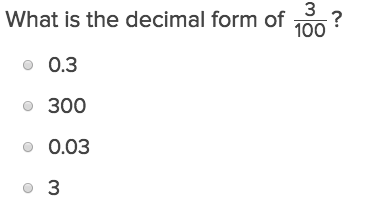Converting decimals to fractions is a lot easier for students once they have worked through this exercise.

## Decimal Fractions and Percents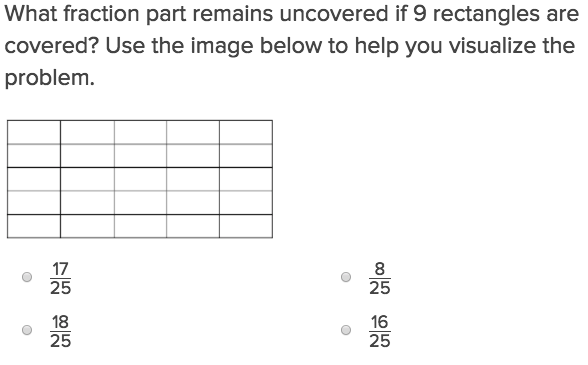Show students how to switch seamlessly back and forth from decimals to fractions to percents with this exercise.

## Area and Unit Fractions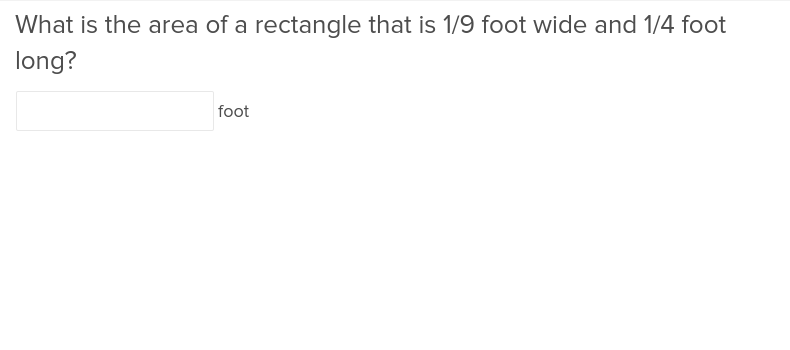Students will understand how to calculate the area of an object with unit fractions as its dimensions after completing this exercise.

## Equivalent Fractions 3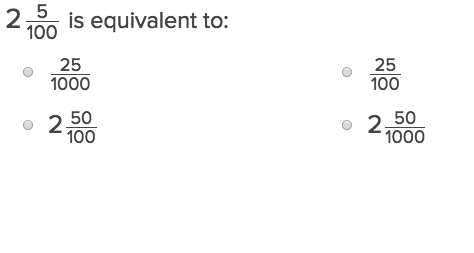Being able to pick out equivalent fractions will be much easier for students who complete this exercise.

## Rectangular Fraction Models and Equivalence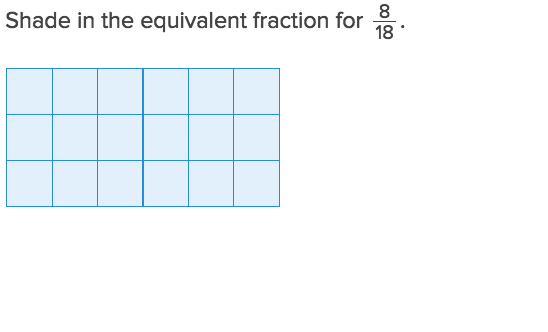If your students need a different way to visualize fractions, show them this exercise on rectangular fraction models and equivalence.

Create new collection

0

### New Collection>

0Items

What could we do to improve Education.com?